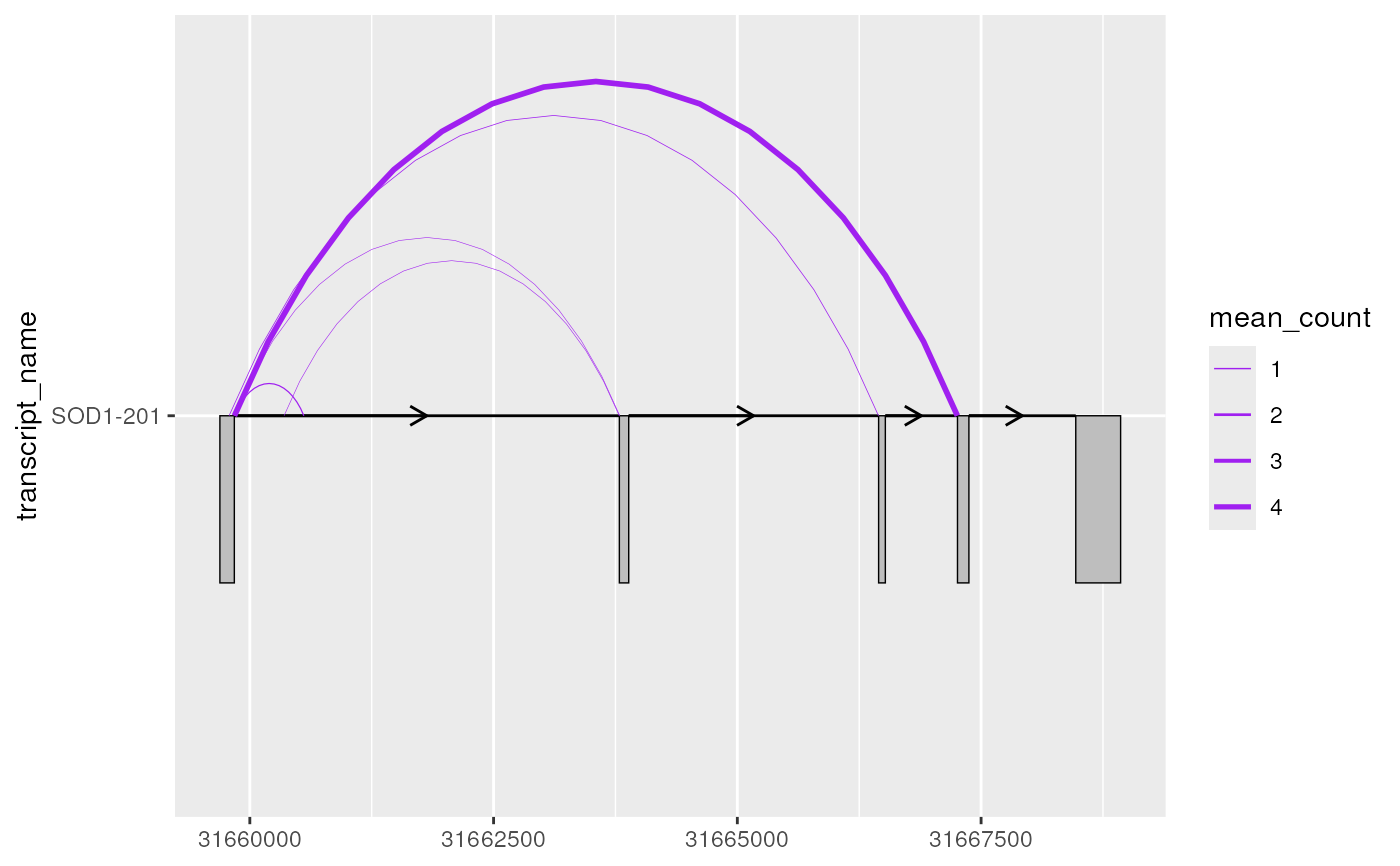geom_junction() draws curves that are designed to represent junction reads from RNA-sequencing data. It can be useful to overlay junction data on transcript annotation (plotted using geom_range()/geom_half_range() and geom_intron()) to understand which splicing events or transcripts have support from RNA-sequencing data.

## Usage

geom_junction(
mapping = NULL,
data = NULL,
stat = "identity",
position = "identity",
junction.orientation = "alternating",
junction.y.max = 1,
angle = 90,
ncp = 15,
na.rm = FALSE,
orientation = NA,
show.legend = NA,
inherit.aes = TRUE,
...
)

## Arguments

mapping

Set of aesthetic mappings created by aes() or aes_(). If specified and inherit.aes = TRUE (the default), it is combined with the default mapping at the top level of the plot. You must supply mapping if there is no plot mapping.

data

The data to be displayed in this layer. There are three options:

If NULL, the default, the data is inherited from the plot data as specified in the call to ggplot().

A data.frame, or other object, will override the plot data. All objects will be fortified to produce a data frame. See fortify() for which variables will be created.

A function will be called with a single argument, the plot data. The return value must be a data.frame, and will be used as the layer data. A function can be created from a formula (e.g. ~ head(.x, 10)).

stat

The statistical transformation to use on the data for this layer, as a string.

position

Position adjustment, either as a string, or the result of a call to a position adjustment function.

junction.orientation

character() one of "alternating", "top" or "bottom", specifying where the junctions will be plotted with respect to each transcript (y).

junction.y.max

double() the max y-value of each junction curve. It can be useful to adjust this parameter when junction curves overlap with one another/other transcripts or extend beyond the plot margins.

angle

A numeric value between 0 and 180, giving an amount to skew the control points of the curve. Values less than 90 skew the curve towards the start point and values greater than 90 skew the curve towards the end point.

ncp

The number of control points used to draw the curve. More control points creates a smoother curve.

na.rm

If FALSE, the default, missing values are removed with a warning. If TRUE, missing values are silently removed.

orientation

The orientation of the layer. The default (NA) automatically determines the orientation from the aesthetic mapping. In the rare event that this fails it can be given explicitly by setting orientation to either "x" or "y". See the Orientation section for more detail.

show.legend

logical. Should this layer be included in the legends? NA, the default, includes if any aesthetics are mapped. FALSE never includes, and TRUE always includes. It can also be a named logical vector to finely select the aesthetics to display.

inherit.aes

If FALSE, overrides the default aesthetics, rather than combining with them. This is most useful for helper functions that define both data and aesthetics and shouldn't inherit behaviour from the default plot specification, e.g. borders().

...

Other arguments passed on to layer(). These are often aesthetics, used to set an aesthetic to a fixed value, like colour = "red" or size = 3. They may also be parameters to the paired geom/stat.

## Value

the return value of a geom_* function is not intended to be directly handled by users. Therefore, geom_* functions should never be executed in isolation, rather used in combination with a ggplot2::ggplot() call.

## Details

geom_junction() requires the following aes(); xstart, xend and y (e.g. transcript name). geom_junction() curves can be modified using junction.y.max, which can be useful when junctions overlap one another/other transcripts or extend beyond the plot margins. By default, junction curves will alternate between being plotted on the top and bottom of each transcript (y), however this can be modified via junction.orientation.

## Examples


library(magrittr)
library(ggplot2)

# to illustrate the package's functionality
# ggtranscript includes example transcript annotation
#> # A tibble: 6 × 8
#>   seqnames  start    end strand type  gene_name transcript_name transcript_biot…
#>   <fct>     <int>  <int> <fct>  <fct> <chr>     <chr>           <chr>
#> 1 21       3.17e7 3.17e7 +      gene  SOD1      NA              NA
#> 2 21       3.17e7 3.17e7 +      tran… SOD1      SOD1-202        protein_coding
#> 3 21       3.17e7 3.17e7 +      exon  SOD1      SOD1-202        protein_coding
#> 4 21       3.17e7 3.17e7 +      CDS   SOD1      SOD1-202        protein_coding
#> 5 21       3.17e7 3.17e7 +      star… SOD1      SOD1-202        protein_coding
#> 6 21       3.17e7 3.17e7 +      exon  SOD1      SOD1-202        protein_coding

# as well as a set of example (unannotated) junctions
sod1_junctions
#> # A tibble: 5 × 5
#>   seqnames    start      end strand mean_count
#>   <fct>       <int>    <int> <fct>       <dbl>
#> 1 chr21    31659787 31666448 +           0.463
#> 2 chr21    31659842 31660554 +           0.831
#> 3 chr21    31659842 31663794 +           0.316
#> 4 chr21    31659842 31667257 +           4.35
#> 5 chr21    31660351 31663789 +           0.324

# extract exons
sod1_exons <- sod1_annotation %>% dplyr::filter(
type == "exon",
transcript_name == "SOD1-201"
)
#> # A tibble: 5 × 8
#>   seqnames  start    end strand type  gene_name transcript_name transcript_biot…
#>   <fct>     <int>  <int> <fct>  <fct> <chr>     <chr>           <chr>
#> 1 21       3.17e7 3.17e7 +      exon  SOD1      SOD1-201        protein_coding
#> 2 21       3.17e7 3.17e7 +      exon  SOD1      SOD1-201        protein_coding
#> 3 21       3.17e7 3.17e7 +      exon  SOD1      SOD1-201        protein_coding
#> 4 21       3.17e7 3.17e7 +      exon  SOD1      SOD1-201        protein_coding
#> 5 21       3.17e7 3.17e7 +      exon  SOD1      SOD1-201        protein_coding

# add transcript_name to junctions for plotting
sod1_junctions <- sod1_junctions %>%
dplyr::mutate(transcript_name = "SOD1-201")

# junctions can be plotted as curves using geom_junction()
base <- sod1_junctions %>%
ggplot2::ggplot(ggplot2::aes(
xstart = start,
xend = end,
y = transcript_name
))

# sometimes, depending on the number and widths of transcripts and junctions
# junctions will go overlap one another or extend beyond the plot margin
base + geom_junction()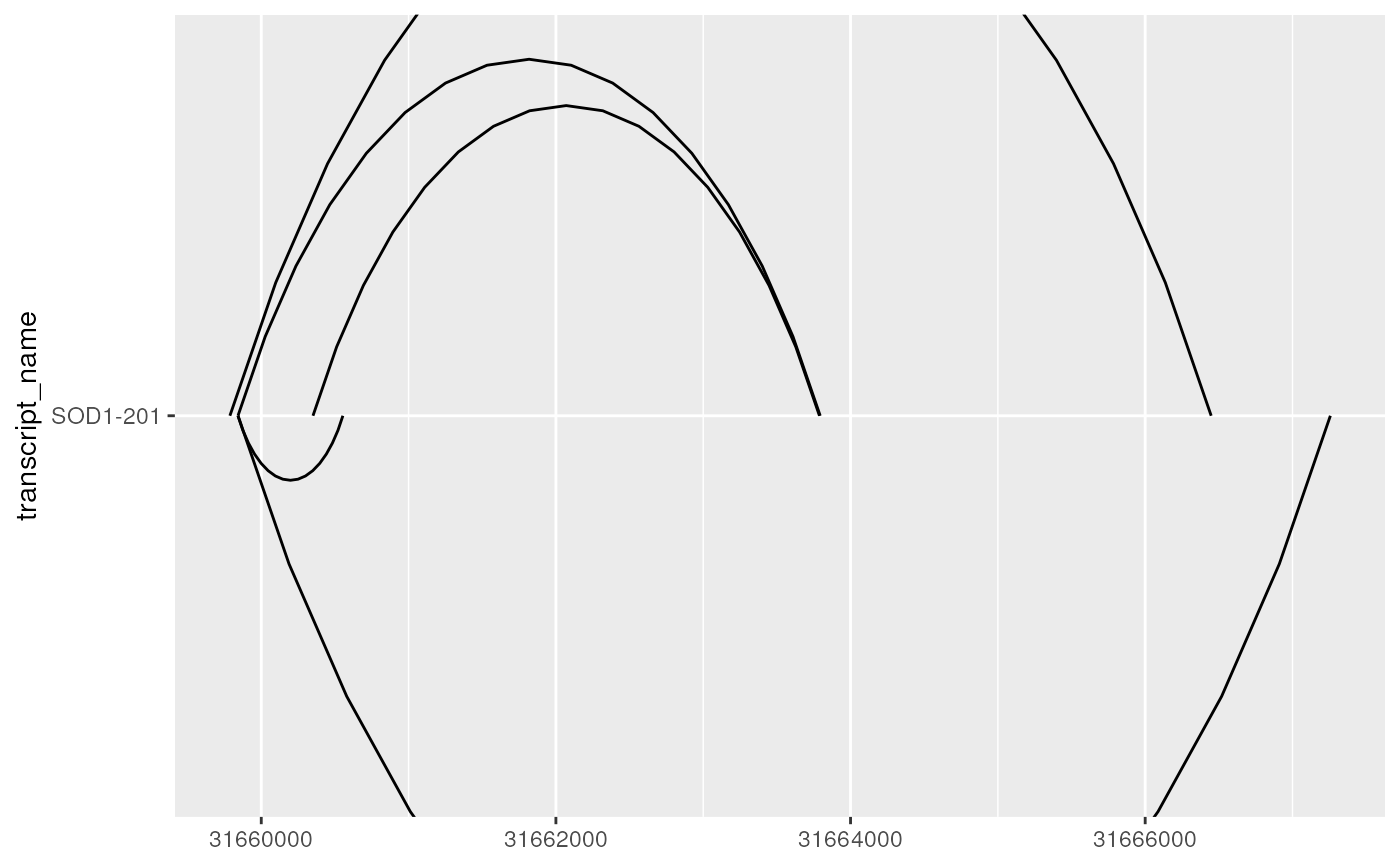# in such cases, junction.y.max can be adjusted to modify the max y of curves
base + geom_junction(junction.y.max = 0.5)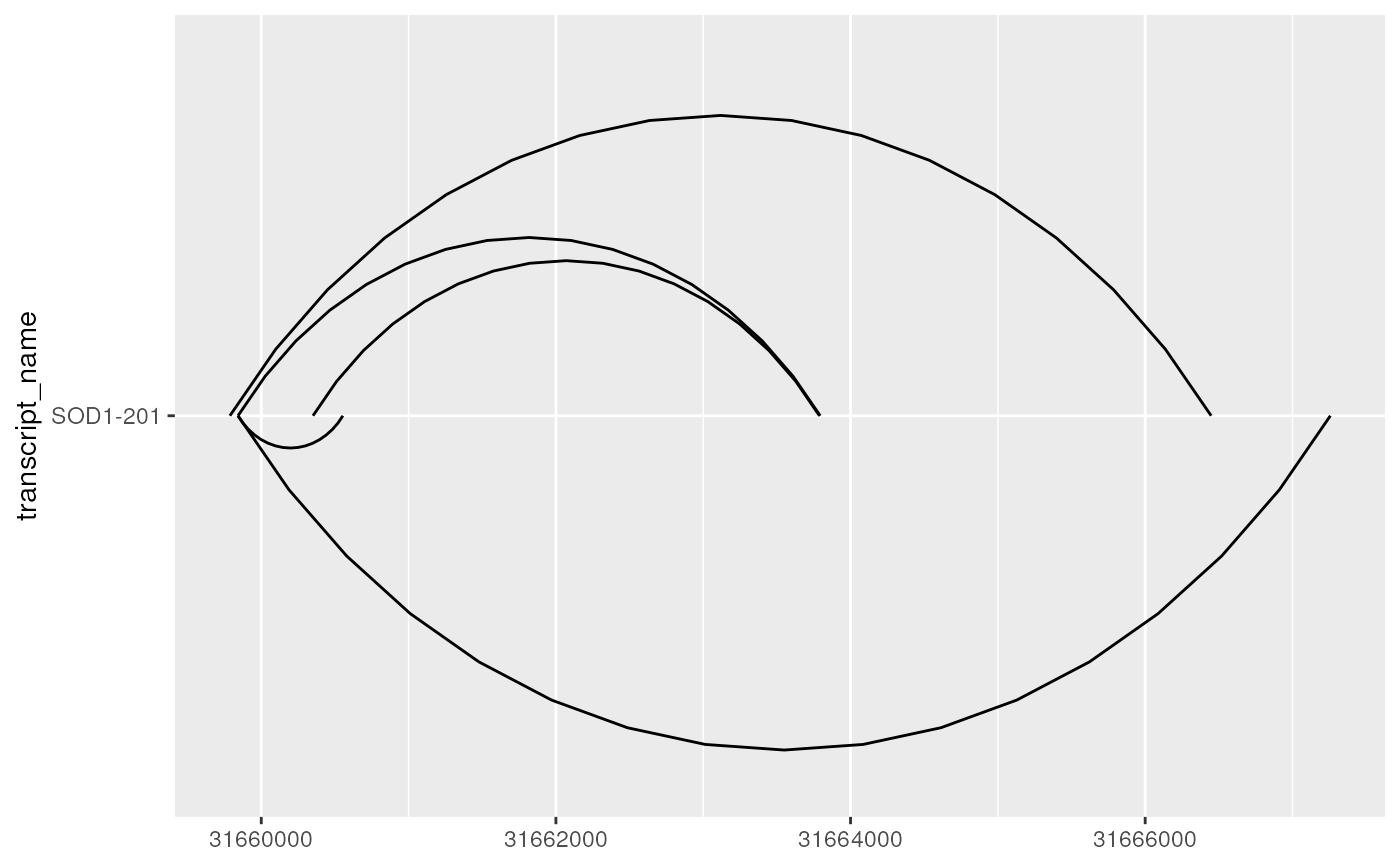# ncp can be used improve the smoothness of curves
base + geom_junction(junction.y.max = 0.5, ncp = 30)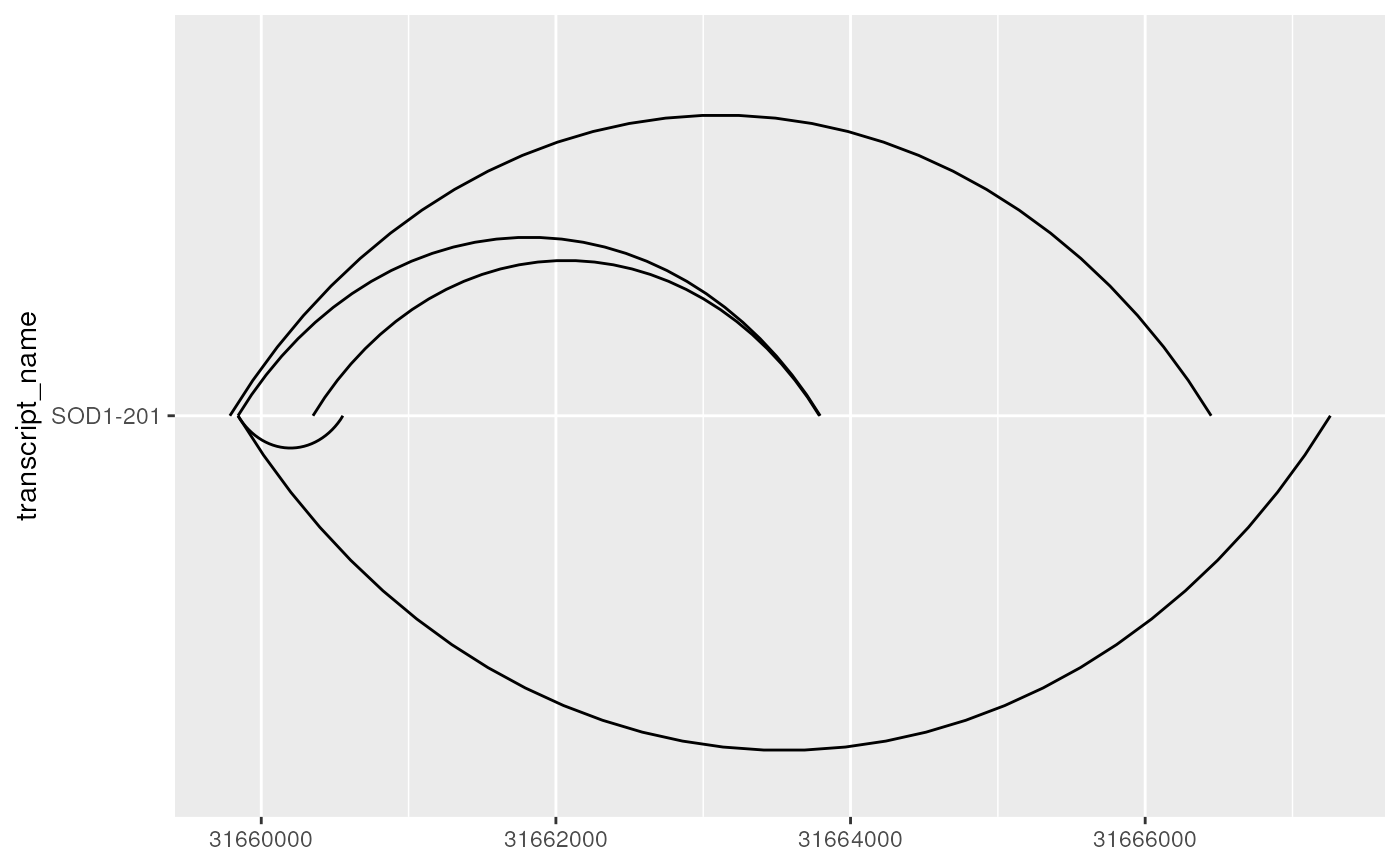# junction.orientation controls where the junction are plotted
# with respect to each transcript
# either alternating (default), or on the top or bottom
base + geom_junction(junction.orientation = "top", junction.y.max = 0.5)base + geom_junction(junction.orientation = "bottom", junction.y.max = 0.5)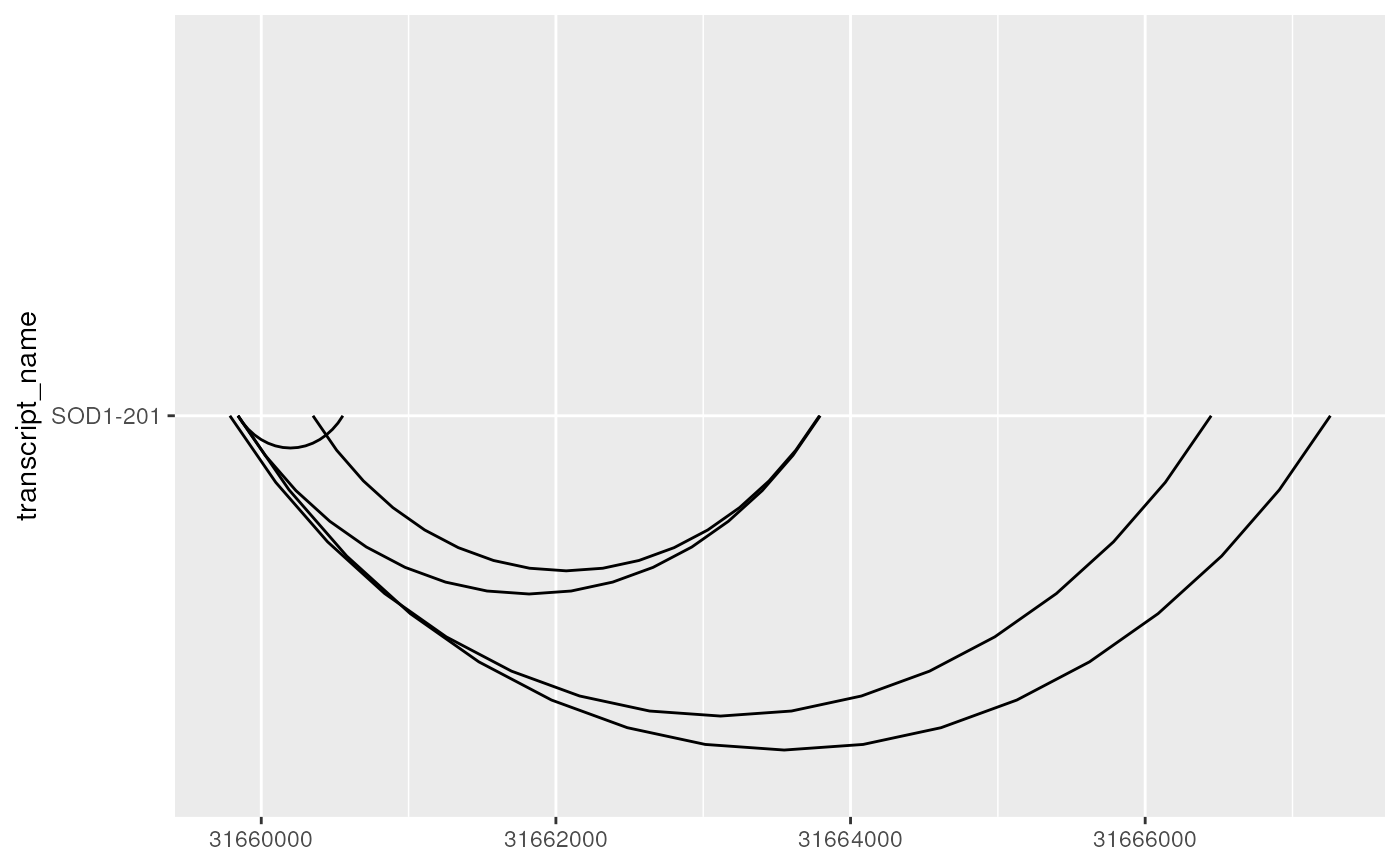# it can be useful useful to overlay junction curves onto existing annotation
# plotted using geom_range() and geom_intron()
base <- sod1_exons %>%
ggplot(aes(
xstart = start,
xend = end,
y = transcript_name
)) +
geom_range() +
geom_intron(
data = to_intron(sod1_exons, "transcript_name")
)

base + geom_junction(
data = sod1_junctions,
junction.y.max = 0.5
)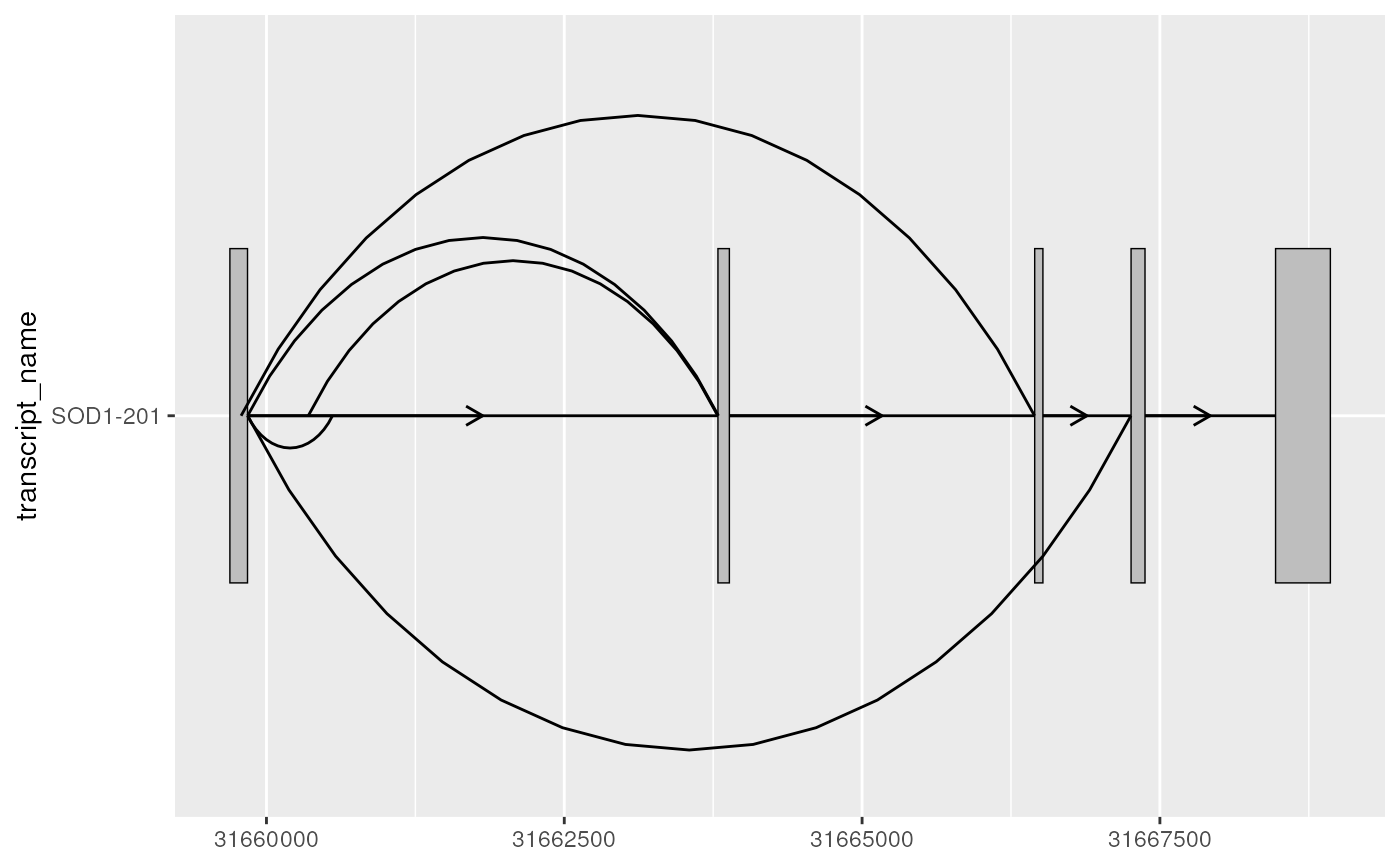# as a ggplot2 extension, ggtranscript geoms inherit the
# the functionality from the parameters and aesthetics in ggplot2
# this can be useful when mapping junction thickness to their counts
base + geom_junction(
data = sod1_junctions,
aes(size = mean_count),
junction.y.max = 0.5,
colour = "purple"
) +
scale_size(range = c(0.1, 1))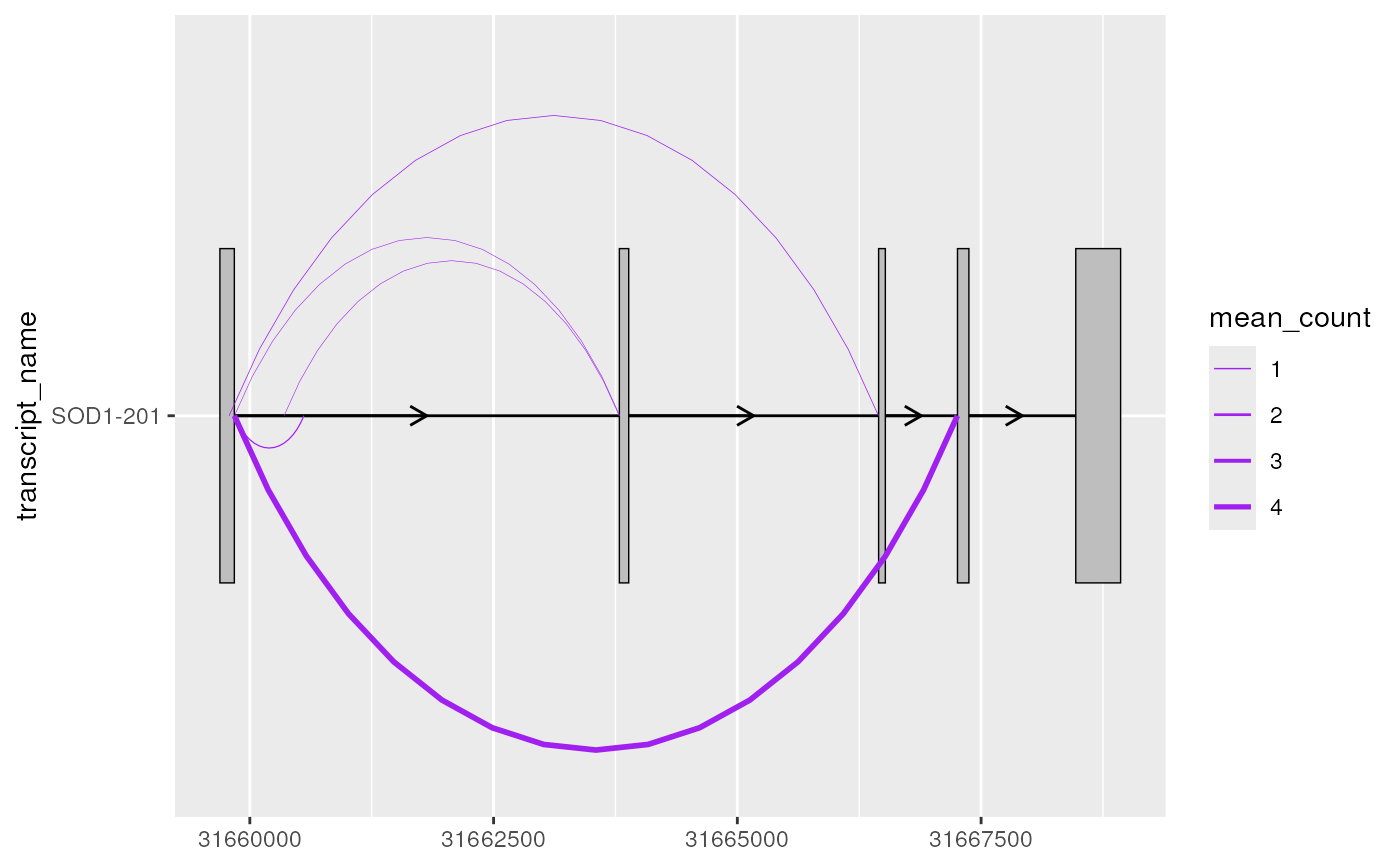# it can be useful to combine geom_junction() with geom_half_range()
sod1_exons %>%
ggplot(aes(
xstart = start,
xend = end,
y = transcript_name
)) +
geom_half_range() +
geom_intron(
data = to_intron(sod1_exons, "transcript_name")
) +
geom_junction(
data = sod1_junctions,
aes(size = mean_count),
junction.y.max = 0.5,
junction.orientation = "top",
colour = "purple"
) +
scale_size(range = c(0.1, 1))